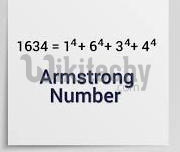# C# Armstrong Number - c# - c# tutorial - c# net

## How to write Armstrong number Program in C# ?

• Armstrong number is a number that is equal to the sum of cubes of its digits.
• For example 0, 1, 153, 370, 371 and 407 are the Armstrong numbers.
• Let's try to understand why 371 is an Armstrong number.
• 371 = (3*3*3)+(7*7*7)+(1*1*1)
• where:
• (3*3*3)=27
• (7*7*7)=343
• (1*1*1)=1
• So:
• 27+343+1=371
• Let's see the C# program to check Armstrong Number.